### Showing videos fromEddie Woo with a total of 4,051 videos07:17
Series & Sequences: Working with a series that has equal sums
1y6m ago05:27
Series & Sequences: Finding a general term from the sum formula
1y6m ago11:05
Completing the Square (1 of 2: How does it help?)
1y6m ago10:24
Partial Sums (1 of 2: Adding up terms in an arithmetic progression)
1y6m ago11:38
The Quadratic Formula (Definition & example question)
1y6m ago08:07
Series & Sequences Introduction (3 of 3: Geometric Progressions)
1y6m ago12:01
Series & Sequences Introduction (2 of 3: Ways to define a sequence)
1y6m ago04:16
Series & Sequences Introduction (1 of 3: Basic definitions)
1y6m ago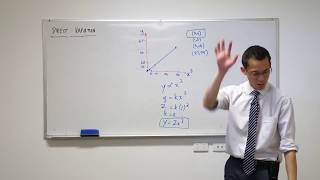03:14
Direct Variation (5 of 5: Solving a problem through the equation)
1y6m ago10:51
Direct Variation (4 of 5: Graphing a relationship with different horizontal axes)
1y6m ago03:22
Direct Variation (3 of 5: Interpreting variation equations)
1y6m ago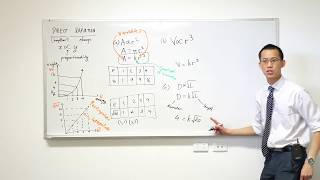08:34
Direct Variation (2 of 5: Forming variation equations)
1y7m ago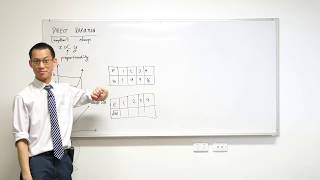09:03
Direct Variation (1 of 5: Relating two changing quantities)
1y7m ago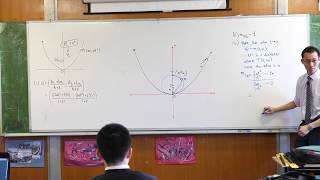07:19
HSC Parametrics Question (2 of 2: Understanding the locus)
1y7m ago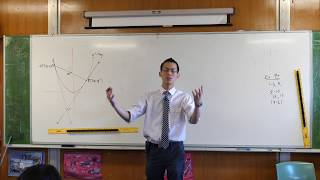10:00
Geometric Proof with a Parabola (Worked Example 2)
1y7m ago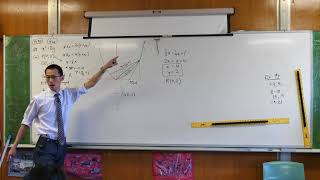11:02
Geometric Proof with a Parabola (Worked Example 1)
1y7m ago09:02
Finding Parabola Given Vertex, Direction & Tangent (2 of 2: Using the discriminant)
1y7m ago07:05
Finding Parabola Given Vertex, Direction & Tangent (1 of 2: Introduction)
1y7m ago11:49
The Reflective Property of Parabolas (3 of 3: Proof)
1y7m ago10:11
The Reflective Property of Parabolas (2 of 3: Relating the relevant angles)
1y7m ago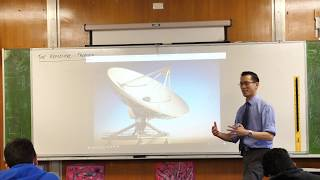07:56
The Reflective Property of Parabolas (1 of 3: Introduction)
1y7m ago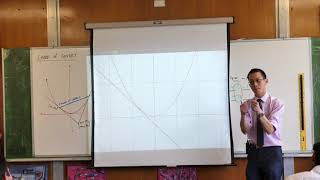05:53
Chord of Contact (3 of 3: Why is it the same as the tangent?)
1y7m ago08:52
Y10 Review Questions (Trigonometry, Simultaneous Equations)
1y7m ago08:10
Parametrics of the Parabola - NESA Reference Sheet Walkthrough (2 of 2)
1y7m ago08:32
Parametrics of the Parabola - NESA Reference Sheet Walkthrough (1 of 2)
1y7m ago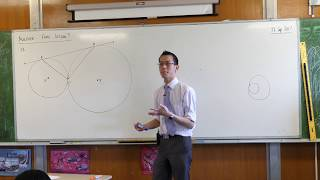06:36
Circle Geometry Proof (Touching circles with a second common tangent)
1y7m ago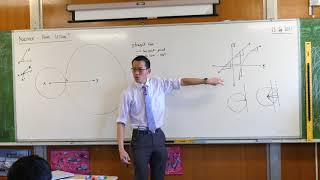11:56
Proof: Centres & point of contact are collinear on touching circles
1y7m ago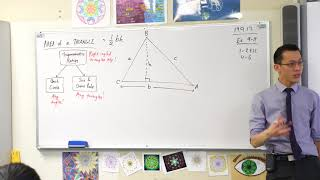07:31
Area of a Triangle (Deriving the trigonometric formula)
1y7m ago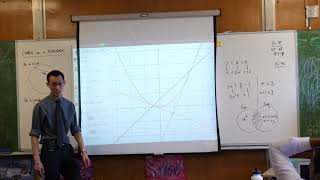05:48
Chords on a Parabola (2 of 2: Exploring the focal chord visually)
1y7m ago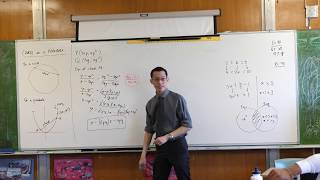07:37
Chords on a Parabola (1 of 2: Deriving its equation)
1y7m ago06:24
What does the parameter mean? (2 of 2: Understanding the geometry)
1y7m ago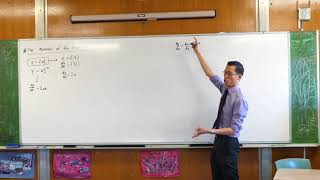07:10
What does the parameter mean? (1 of 2: Exploring the derivatives)
1y7m ago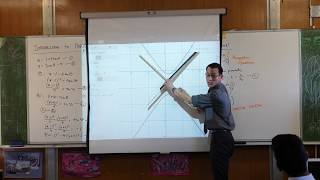03:28
Parametric equations → Cartesian equation (2 of 2: What's the graph?)
1y7m ago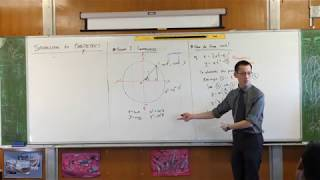11:12
Parametric equations → Cartesian equation (1 of 2: Manipulating with trigonometric identities)
1y7m ago04:23
Introduction to Parametrics (2 of 4: How to eliminate a parameter)
1y7m ago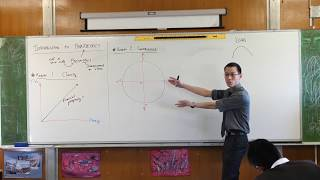08:06
Introduction to Parametrics (1 of 4: Clarity & convenience)
1y7m ago09:43
Cosine Rule (1 of 2: Stating & re-arranging the formula)
1y7m ago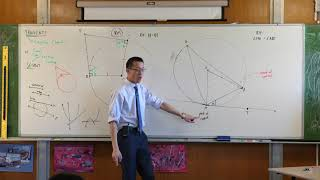11:24
Tangents of Circles (4 of 4: Angle in the alternate segment)
1y7m ago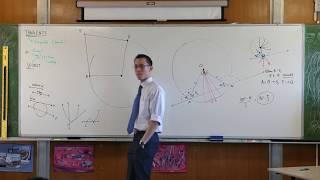05:10
Tangents of Circles (3 of 4: Tangents from an external point)
1y7m ago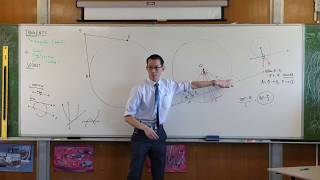07:00
Tangents of Circles (2 of 4: Tangent perpendicular to radius)
1y7m ago04:06
Applying the Sine Rule (2 of 2: Finding an obtuse angle)
1y7m ago05:55
Applying the Sine Rule (1 of 2: Finding an acute angle)
1y7m ago05:18
Solving Trigonometric Equations with the Unit Circle
1y7m ago07:07
Y10 Review Questions (Solving trigonometric equations with quadrants)
1y7m ago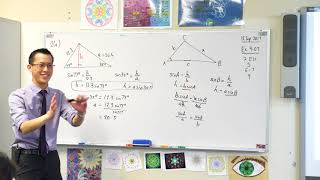08:40
The Sine Rule (2 of 2: Generalising the formula)
1y7m ago06:38
The Sine Rule (1 of 2: Introduction through example)
1y7m ago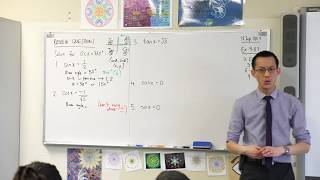12:21
Y10 Review Questions (Solving simple trigonometric equations)
1y7m ago09:58
Binomial Theorem question: "Coefficient of x³ is double the coefficient of x²"
1y7m ago07:21
Working with a Quadratic Equation involving Algebraic Coefficients
1y7m ago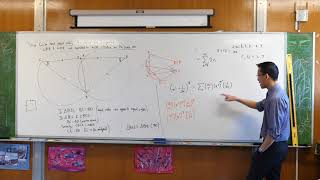08:05
Equal Overlapping Circles Question (2 of 2: Finding equal lengths)
1y7m ago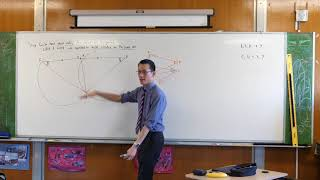12:27
Equal Overlapping Circles Question (1 of 2: Identifying congruent triangles)
1y7m ago11:27
Intro to Trigonometric Functions (2 of 2: Plotting the graph)
1y7m ago09:44
Intro to Trigonometric Functions (1 of 2: Angles of any magnitude)
1y7m ago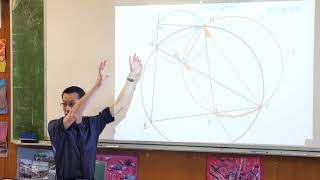06:48
Tough Circle Geometry Question (2 of 2: Proving points collinear)
1y7m ago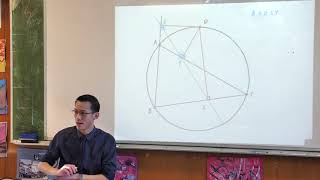07:06
Tough Circle Geometry Question (1 of 2: Set up & initial arguments)
1y7m ago